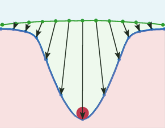## Introduction

The Earth as a whole responses to external forces as an elastic body while the ocean response far from the shore takes the shape of equipotential surface. For this reason we observe ocean tides: relative redistribution of ocean with respect to the litisphere. Due to viscosity response the ocean response deviates fom the equipotential surface, especially in shallow seas. Ocean mass re-distribution due to tides causes crustal defromation called ocean tidal loading.

Tides can be represented as a sum of quasi-harmonic consituents that are characterized by frequency, angualar accelration, phase, sine, and cosine amplitudes. The last three parameters depend on site coordinates.

Tidal constituents consist of short-periodic tides with frequencies around the diurnal frequency and its harmonics, long-periodic (or zonal) with preiods from 18.6 years to 5 days, and several pole tide consituets. The pole tide is caused by changes in the centrifugial potential due to polar motion. Other tides are caused by gravitational attraction of the Moon and the Sun. The vertical dispalcements typically have peak to peak variations around 1 cm, although there are areas where they can be as large as 10 cm.

On 2014.06.17 the service of non-tidal atmospheric pressure loading was established by Leonid Petrov. The service is based on the spherical harmonics transform of the bottom pressure exerted by the ocean tide accurding to several tidal models. In general, tides are split into
1. gravity tides described by the tidal model that are based on assimilation of satellite altimiter data and the underlying numerical model that is a result of solving hydrodynamics equiations. Gravity tides consist of a number of quasi-harmonics consituents: A_c cos ( P_c + Ω t + 1/2 α t2 ) + A_s sin ( P_s + Ω t + 1/2 α t2 )   where α is the angular acceleration.

2. long periodic tides described by the so-called equillibrium tidal model that assumes ocean water for these consituents follows the equipotential surface with correction for total ocean mass conservation and self-loading, i.e. considering crustal deformation of the ocean bottom. Deviation of long-periodic tides from the equllibrium is not large. So,e gravity tides model include several (but not all) long periodic models.

3. pole tides described by the equillibrium tidal response and a harmonic model of polar motion. Pole motion and therefore, changes in the centrifugial potential over [1984, 2014] are approximated as a sum of three harmonic component with periods 365.24 days (annual), 431.18 days, and 445.41 days (Chandler period) with rms of resoduals 0.029 mas. Therefore, pole tides can be considered using the same formalism as gravity tides.

### The survice provides

• Precomputed coefficients of harmonic variations of 3D displacements 1272 VLBI, GPS, SLR, and DORIS stations. Displacements are in HARPOS format.

• Precomputed coefficients of harmonic variations of 3D displacements of ocean tidal loading at a 2′ × 2′ global grid. Displacements are in NETCDF format.

• Site displacements: 17 gravity tides according to GOT 4.10c model for gravity tides.
• Site displacements: 44 gravity tides according to FES2014b model for gravity tides, including 7 long period tide.
• Site displacements: 3 pole tides and 1 long-periodic gravity tide according to the equillibrium tide.
• Site displacements: 3 pole tides and 9 long-periodic gravity tide according to the equillibrium tide.
• On-demand online computation of tidal ocean loading for an arbitrary point at the Earth. You can order computation of the time series for the stations of your interest. You need to prepare a station file in plain ascii that has four columns separated by one or more blanks:

Station_name    X-coordinate    Y-coordinate    Z-coordinate

Station name should have no more than 8 characters. X,Y,Z are Cartesian coordinates of the station of interest in a crust-fixed coordinates system. Units are meters. Here is an example.

Model:  GOT-4.8 GOT-4.10C FES2012 FES2014B EQUIL01 EQUIL02
Frame:  Center of mass Center of figure
Station file:

Results of on-demand computations are accessible from here.

## References

1. Darwin, G.H., On variations in the vertical due to elasticity of the Earth's surface, Phil. Mag., Ser. 5, col. 14, N. 90, 409--427, 1882.
2. Farrell, W.E, Deformation of the Earth by Surface Loads, Rev. Geophys. and Spac. Phys., vol. 10(3), pp. 751--797, 1972.

## Acknowledgment

This work was supported by NASA
Earth Surface & Interior program, grant NNX12AQ29G.

This page was prepared by Leonid Petrov ()